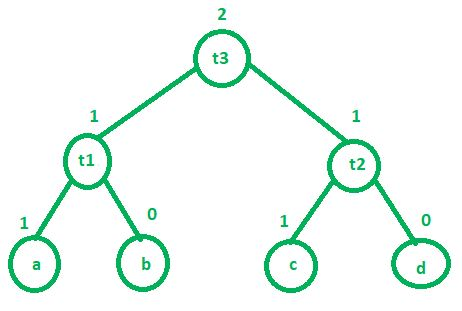# Labeling Algorithm in Compiler Design

Labeling algorithm is used by compiler during code generation phase. Basically, this algorithm is used to find out how many registers will be required by a program to complete its execution. Labeling algorithm works in bottom-up fashion. We will start labeling firstly child nodes and then interior nodes. Rules of labeling algorithm are:

Traverse the control flow graph of the program and assign a label to the first instruction of each basic block.

For each jump or branch instruction, assign a label to the target basic block if it does not already have a label.

Repeat step 2 until all target basic blocks have labels.

Generate the code for each instruction, using the labels to specify the target addresses for jump and branch instructions.

The labeling algorithm ensures that each basic block has a unique label and that the labels are assigned in the correct order to ensure that all target basic blocks have labels.

### The advantages of the labeling algorithm include:

1. Efficient code generation: The labeling algorithm ensures that the code generated for jump and branch instructions is correct and efficient.
2. Flexibility: The labeling algorithm can be adapted to a wide range of programming languages and architectures.
3. Easy to implement: The labeling algorithm is a simple and easy-to-implement technique.

### However, the labeling algorithm also has some disadvantages:

1. Limited optimization: The labeling algorithm does not optimize the code generated for jump and branch instructions.
2. Increased code size: The labels generated by the labeling algorithm increase the size of the code.

In summary, the labeling algorithm is a technique used in compiler design to generate labels for instructions during code generation. It ensures that the code generated for jump and branch instructions is correct and efficient, but it does not optimize the code and can increase the size of the code.

1. If ‘n’ is a leaf node –
• a. If ‘n’ is a left child then its value is 1.
• b. If ‘n’ is a right child then its value is 0.
2. If ‘n’ is an interior node – Lets assume L1 and L2 are left and right child of interior node respectively.
• a. If L1 == L2 then value of ‘n’ is L1 + 1 or L2 + 1
• b. If L1 != L2 then value of ‘n’ is MAX(L1, L2)

Example: Consider the following three address code:

```t1 = a + b
t2 = c + d
t3 = t1 + t2 ```Above three address code will require maximum 2 registers to complete its execution. There is a function called getregister() which is used by compiler to decide where the result will get stored. There are 4 cases of this function which are as follows:

1. If there is a register R which is not holding multiple values then we can use this register to store the value of our result (in the above example, we can store t3 in R, provided that current value in R is not being used anywhere in the program.).
2. If first condition is not satisfied then compiler will search for any empty register to store the value of our result (t3).
3. If there are no empty registers then swap the contents of any registers into the memory and store the result (t3) in that register, provided that those contents do not have any next use.
4. If all the 3 conditions do not hold then store the result in any free memory location.